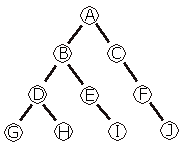## Binary Trees

### What is a binary tree?

A binary tree is a graphical representation of a series of numbers or symbols. Essentially this page will help you figure out how to traverse (move through) a binary tree (there’s three ways) and also how to identify several different types of binary trees.

Note: For simplicity sake, we will be using the binary tree below for all examples.### Traversing

Traversing a binary tree just means putting the values of a binary tree in a certain order based on their positions in the tree. On WYSE tests, you will be given a binary tree and asked what value you should get if you traverse a tree a certain way. The three ways to traverse a tree are: in-order, pre-order, post-order.

In-order: Start at the left most child, move to the root of that child, move to the right child of that root, continue. For this method, we would start at G because it is the left-most child of the tree, move to its root, which is D, then to the right child, which is H. From there we move to B because it is the root of the GDH tree, then down to E and I because it is the right side of the GDHB tree. We’ll keep doing this, and at the end we’ll get a value of GDHBEiACFJ.

Pre-order: Start at the root, then move to the left child, then the right child. We’ll start at the root of the entire tree, which is A, then move to B since it’s the left child, but instead of going to C we have to do B’s children, going left to right. Eventually we’ll end up with: ABDGHEICFJ.

Post-order: Start at the left-most child, then the right-most child, then the root. Just like the in-order traversal, we’ll start at G, but instead of moving to its root D, we’ll go to the right child of H, then D. We’ll continue this and return a value of: GHDIEBJFCA.

Want an easy way to remember these? The in-, pre-, and post- all refer to the position of when the root value comes in. In pre-, root comes first. In in-, root is in the middle. In post-, root is last. I’ll redefine the three traversals using this method and it should all be extremely clear:

In-order: left, root, right

Pre-order: root, left, right

Post-order: left, right, root

### Different types of trees

Sometimes you’ll be given a tree and asked to identify it. Here’s a few defintions of common trees:

Expression tree: All the nodes (the circles) are expressions…like +, -, *, /, etc.

Min heap: For every node that only has one child, that child must be in the left position (you can’t have a node in a right position if it’s the only child)

Search tree: Left child must be smaller than the parent node. The right child can be bigger, but the left cannot.

Complete binary tree: Every node that has children has two children — no root has only one child

Previous: SortingPrefix, Infix, Postfix